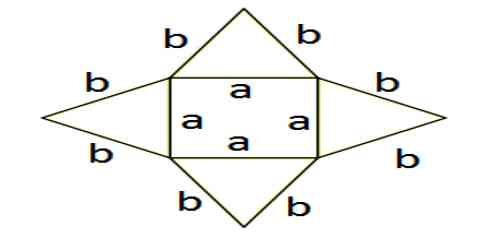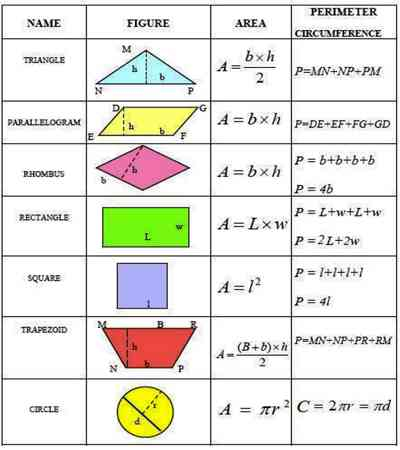Other

# Formula on MensurationFormula on Mensuration

Mensuration is the branch of mathematics which deals with the study of Geometric shapes, their area, volume and related parameters.

It is based on the use of algebraic equations and geometric calculations to provide measurement data regarding the width, depth and volume of a given object or group of objects. While the measurement results obtained by the use of mensuration are estimates rather than actual physical measurements, the calculations are usually considered very accurate.

Prism

(i) Area of the lateral faces of a right prism = perimeter of the base × height.

(ii) Area of the whole surface of a right prism = Area of the lateral faces + 2 × area of the base.

(iii) Volume of a right prism = area of the base × height.

Pyramid

(i) Area of the lateral faces of a right pyramid = ½ × perimeter of the base x slant height.

(ii) Area of whole surface of a right pyramid = area of the lateral faces + area of the base.

(iii) Volume of a right pyramid = 1/3 × area of the base × height.

Tetrahedron

If a be the length of an edge of a regular tetrahedron then

(i) the area of its slant sides = 3√3/4 a2 sq. units;

(ii) the area of its whole surface = √3a2 sq. units

(iii) the volume of the tetrahedron = √2/12 a3 cu. units.

Mensuration Formulas for Rectangle

Area of Rectangle = Length × Breadth.

Perimeter of a Rectangle = 2 × (Length + Breadth)

Length of the Diagonal = √(Length2 + Breadth2)Mensuration Formulas for Square

Area of a Square = Length × Length = (Length)2

Perimeter of a square = 4 × Length

Length of the Diagonal = √2 × Length

Trapezium

Area = 1/2h(a+b)

Perimeter = Sum of all sides

Rhombus

Area = d1 × d2/2

Perimeter = 4l

Area =1/2 × Diagonal × (Sum of offsets)

Kite

Area  = d1×d2/2

Perimeter  = 2 × Sum on non-adjacent sides

Circle

Area =  πr^2 or πd^2/4

Circumference = 2πr  or πd

Area of sector of a circle = (θπr^2 )/360

Information Source-